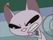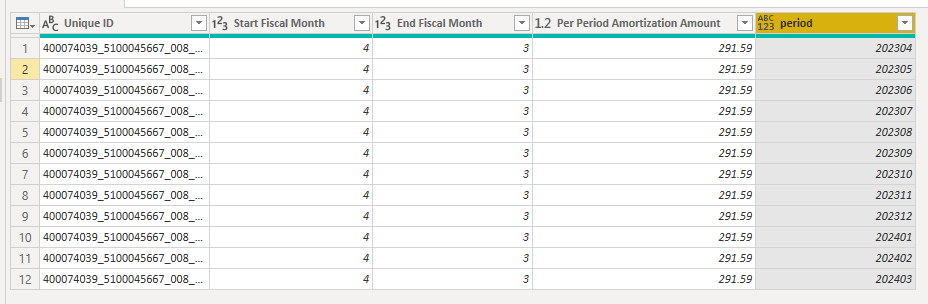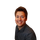cancel
Showing results for
Did you mean:Helper IV

## Generating a list of numbers starting from specified value and restarting after reaching 12

I'm trying to address a challenge most of which is solved by @BA_Pete here: Adding columns or rows for missing data - Microsoft Power BI Community

I think I have an approach but I need to generate a list of month numbers that starts at a value specified in the [Start Fiscal Month] column, increments up to 12, and then starts at 1 again until the total number of iterations reaches the number of months specified.

 Start Fiscal Month Start Fiscal Year End Fiscal Month End Fiscal Year Month List 4 2023 3 2024 4 4 2023 3 2024 5 4 2023 3 2024 6 4 2023 3 2024 7 4 2023 3 2024 8 4 2023 3 2024 9 4 2023 3 2024 10 4 2023 3 2024 11 4 2023 3 2024 12 4 2023 3 2024 1 4 2023 3 2024 2 4 2023 3 2024 3

I've looked at List.Generate and List.Repeat but not been able to get to what I need.

1 ACCEPTED SOLUTIONSuper User

--UPDATED to handle multi-year amortisation--

``````let
Source = Table.FromRows(Json.Document(Binary.Decompress(Binary.FromText("Jci7DcAgDIThXVxH6PyCeIZ0aRHy/ltgkuJ0n/45yQAMg0Y6F817Hwnc+bx0kVpEYylZTSB64k8/9+Xg5kFrbQ==", BinaryEncoding.Base64), Compression.Deflate)), let _t = ((type nullable text) meta [Serialized.Text = true]) in type table [#"Unique ID" = _t, #"Amount in local currency" = _t, #"Start Fiscal Month" = _t, #"Start Fiscal Year" = _t, #"End Fiscal Month" = _t, #"End Fiscal Year" = _t, #"Amortization Period in months" = _t, #"Per Period Amortization Amount" = _t]),
chgTypes = Table.TransformColumnTypes(Source,{{"Amount in local currency", type number}, {"Start Fiscal Month", Int64.Type}, {"Start Fiscal Year", Int64.Type}, {"End Fiscal Month", Int64.Type}, {"End Fiscal Year", Int64.Type}, {"Amortization Period in months", Int64.Type}, {"Per Period Amortization Amount", type number}}),

expandRowNumber,
"period",
each let
year = Text.From([Start Fiscal Year] + (Number.RoundUp(([Start Fiscal Month] + [rowNumber]) / 12) - 1)),
month = Text.PadStart(Text.From(Number.Mod([Start Fiscal Month] + [rowNumber] - 1, 12) + 1), 2, "0")
in
Number.From(Text.Combine({year, month}))),

remOthCols = Table.SelectColumns(addPeriod,{"Unique ID", "Start Fiscal Month", "End Fiscal Month", "Per Period Amortization Amount", "period"})
in
remOthCols``````

Output:Pete

Now accepting Kudos! If my post helped you, why not give it a thumbs-up?

Proud to be a Datanaut!

10 REPLIES 10Memorable Member

Given the first four columns of your posted table (actually just need the first two columns, my approach would be to generate an appropriate List of the fiscal month numbers, and then append that to your original table.

The basic formula to generate that list would be: List.Transform(List.Numbers(Source[Start Fiscal Month]{0},12),
each Number.Mod(_ -1,12) + 1)

In code, with the table stored in Excel

``````let

//Change next line to reflect your actual data source
Source = Excel.CurrentWorkbook(){[Name="Table4"]}[Content],

//Create list of Fiscal months depending on the Start Fiscal Month column
#"Fiscal Months" = List.Transform(List.Numbers(Source[Start Fiscal Month]{0},12),
each Number.Mod(_ -1,12) + 1),

//add that to the original table
#"Add Fiscal Months Column" = Table.FromColumns(
Table.ToColumns(Source) & {#"Fiscal Months"},
type table[Start Fiscal Month=Int64.Type, Start Fiscal Year=Int64.Type, End Fiscal Month=Int64.Type, End Fiscal Year=Int64.Type,Month List=Int64.Type]
)
inSolution Sage

Hello, @vgeldbr I'd also play with List.Sort( {1..12} ) and it's optional comparisonCriteria parameter as an alternative solution...

``````let
Source = Table.FromRows(Json.Document(Binary.Decompress(Binary.FromText("i45WMlHSUTIyMDIGUsYQpolSbCwA", BinaryEncoding.Base64), Compression.Deflate)), let _t = ((type nullable text) meta [Serialized.Text = true]) in type table [#"Start Fiscal Month" = _t, #"Start Fiscal Year" = _t, #"End Fiscal Month" = _t, #"End Fiscal Year" = _t]),
#"Changed Type" = Table.TransformColumnTypes(Source,{{"Start Fiscal Month", Int64.Type}}),
result =
#"Changed Type",
"Month List",
(x) =>
let
m_list = List.Buffer({1..12})
in List.LastN(m_list, 13 - x[Start Fiscal Month]) & List.FirstN(m_list, x[Start Fiscal Month] - 1)
),
expand_m = Table.ExpandListColumn(result, "Month List")
in
expand_m``````Super User

This is the approach I was looking at to resolve your other post. Clearly I wasn't quick enough.

Pete

Now accepting Kudos! If my post helped you, why not give it a thumbs-up?

Proud to be a Datanaut!Helper IV

Thanks @BA_Pete . I did not want to burden you in the original question on the finer details as your approach is what will work and your solution would work for anybody not constrained by a wierd 544 financial year model. Any guidance welcome here or there!Super User

No problem.

Try this:

``````let
Source = Table.FromRows(Json.Document(Binary.Decompress(Binary.FromText("i45WMjEwMDA3MTC2jDc1BDJNTM3MzOMNDCzivYOUdJSMTSwt9QyNgCwTIDYyMDIGCUKYIBGwlJGloZ6ppVJsLAA=", BinaryEncoding.Base64), Compression.Deflate)), let _t = ((type nullable text) meta [Serialized.Text = true]) in type table [#"Unique ID" = _t, #"Amount in local currency" = _t, #"Start Fiscal Month" = _t, #"Start Fiscal Year" = _t, #"End Fiscal Month" = _t, #"End Fiscal Year" = _t, #"Amortization Period in months" = _t, #"Per Period Amortization Amount" = _t]),
chgTypes = Table.TransformColumnTypes(Source,{{"Amount in local currency", type number}, {"Start Fiscal Month", Int64.Type}, {"Start Fiscal Year", Int64.Type}, {"End Fiscal Month", Int64.Type}, {"End Fiscal Year", Int64.Type}, {"Amortization Period in months", Int64.Type}, {"Per Period Amortization Amount", type number}}),

expandRowNumber,
"period",
each let
year = Text.From(if [Start Fiscal Month] + [rowNumber] > 12 then [End Fiscal Year] else [Start Fiscal Year]),
month = Text.PadStart(Text.From(Number.Mod([Start Fiscal Month] + [rowNumber] - 1, 12) + 1), 2, "0")
in
Number.From(Text.Combine({year, month}))
),

remOthCols = Table.SelectColumns(addPeriod,{"Unique ID", "Start Fiscal Month", "End Fiscal Month", "Per Period Amortization Amount", "period"})
in
remOthCols``````

To get this output:Pete

Now accepting Kudos! If my post helped you, why not give it a thumbs-up?

Proud to be a Datanaut!Super User

--UPDATED to handle multi-year amortisation--

``````let
Source = Table.FromRows(Json.Document(Binary.Decompress(Binary.FromText("Jci7DcAgDIThXVxH6PyCeIZ0aRHy/ltgkuJ0n/45yQAMg0Y6F817Hwnc+bx0kVpEYylZTSB64k8/9+Xg5kFrbQ==", BinaryEncoding.Base64), Compression.Deflate)), let _t = ((type nullable text) meta [Serialized.Text = true]) in type table [#"Unique ID" = _t, #"Amount in local currency" = _t, #"Start Fiscal Month" = _t, #"Start Fiscal Year" = _t, #"End Fiscal Month" = _t, #"End Fiscal Year" = _t, #"Amortization Period in months" = _t, #"Per Period Amortization Amount" = _t]),
chgTypes = Table.TransformColumnTypes(Source,{{"Amount in local currency", type number}, {"Start Fiscal Month", Int64.Type}, {"Start Fiscal Year", Int64.Type}, {"End Fiscal Month", Int64.Type}, {"End Fiscal Year", Int64.Type}, {"Amortization Period in months", Int64.Type}, {"Per Period Amortization Amount", type number}}),

expandRowNumber,
"period",
each let
year = Text.From([Start Fiscal Year] + (Number.RoundUp(([Start Fiscal Month] + [rowNumber]) / 12) - 1)),
month = Text.PadStart(Text.From(Number.Mod([Start Fiscal Month] + [rowNumber] - 1, 12) + 1), 2, "0")
in
Number.From(Text.Combine({year, month}))),

remOthCols = Table.SelectColumns(addPeriod,{"Unique ID", "Start Fiscal Month", "End Fiscal Month", "Per Period Amortization Amount", "period"})
in
remOthCols``````

Output:Pete

Now accepting Kudos! If my post helped you, why not give it a thumbs-up?

Proud to be a Datanaut!Helper IV

Thanks @BA_Pete , this works perfectly. Thanks everyone for the ideas all of which have helped me a learn that bit more about Power Query techniques!Super User

Insert this step where #"Changed Type" should be replaced with your previous step

``= Table.FromColumns(Table.ToColumns(#"Changed Type") & {List.Generate(()=>[x=Table.FirstValue(#"Changed Type"),i=0], each [i]<Table.RowCount(#"Changed Type"), each [i=[i]+1, x = if [x]=12 then 1 else [x]+1], each [x])},Table.ColumnNames(#"Changed Type")&{"Month List"})``Helper IV

@Vijay_A_Verma please could you elaborate? Are you assuming the table in my example exists with those exact columns? When I insert into my real query which has other columns I get totally wierd results and I don't really understand the approach you've taken (though I'm trying).Resolver IV

You could try the solution offered here:

https://community.powerbi.com/t5/Desktop/Restart-count-in-column/m-p/43546

Haven't tried it myself, but I think that you can input the value of column StartFiscalMonth as the startposition in the index step.